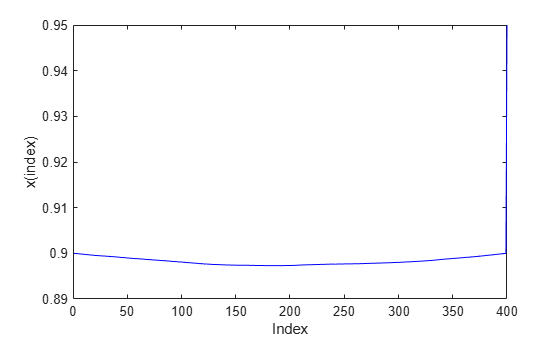# Quadratic Programming with Bound Constraints: Problem-Based

This example shows how to formulate and solve a scalable bound-constrained problem with a quadratic objective function. The example shows the solution behavior using several algorithms. The problem can have any number of variables; the number of variables is the scale. For the solver-based version of this example, see Quadratic Minimization with Bound Constraints.

The objective function, as a function of the number of problem variables n, is

`$2\sum _{i=1}^{n}{x}_{i}^{2}-2\sum _{i=1}^{n-1}{x}_{i}{x}_{i+1}-2{x}_{1}-2{x}_{n}.$`

### Create Problem

Create a problem variable named `x` that has 400 components. Also, create an expression named `objec` for the objective function. Bound each variable below by 0 and above by 0.9, except allow ${\mathit{x}}_{\mathit{n}}$ to be unbounded.

```n = 400; x = optimvar('x',n,'LowerBound',0,'UpperBound',0.9); x(n).LowerBound = -Inf; x(n).UpperBound = Inf; prevtime = 1:n-1; nexttime = 2:n; objec = 2*sum(x.^2) - 2*sum(x(nexttime).*x(prevtime)) - 2*x(1) - 2*x(end);```

Create an optimization problem named `qprob`. Include the objective function in the problem.

`qprob = optimproblem('Objective',objec);`

Create options that specify the `quadprog` `'trust-region-reflective'` algorithm and no display. Create an initial point approximately centered between the bounds.

```opts = optimoptions('quadprog','Algorithm','trust-region-reflective','Display','off'); x0 = 0.5*ones(n,1); x00 = struct('x',x0);```

### Solve Problem and Examine Solution

Solve the problem.

`[sol,qfval,qexitflag,qoutput] = solve(qprob,x00,'options',opts);`

Plot the solution.

```plot(sol.x,'b-') xlabel('Index') ylabel('x(index)')```Report the exit flag, the number of iterations, and the number of conjugate gradient iterations.

```fprintf('Exit flag = %d, iterations = %d, cg iterations = %d\n',... double(qexitflag),qoutput.iterations,qoutput.cgiterations)```
```Exit flag = 3, iterations = 19, cg iterations = 1668 ```

There were a lot of conjugate gradient iterations.

### Adjust Options for Increased Efficiency

Reduce the number of conjugate gradient iterations by setting the `SubproblemAlgorithm` option to `'factorization'`. This option causes the solver to use a more expensive internal solution technique that eliminates conjugate gradient steps, for a net overall savings of time in this case.

```opts.SubproblemAlgorithm = 'factorization'; [sol2,qfval2,qexitflag2,qoutput2] = solve(qprob,x00,'options',opts); fprintf('Exit flag = %d, iterations = %d, cg iterations = %d\n',... double(qexitflag2),qoutput2.iterations,qoutput2.cgiterations)```
```Exit flag = 3, iterations = 10, cg iterations = 0 ```

The number of iterations and of conjugate gradient iterations decreased.

### Compare Solutions With `'interior-point'` Solution

Compare these solutions with that obtained using the default `'interior-point'` algorithm. The `'interior-point'` algorithm does not use an initial point, so do not pass `x00` to `solve`.

```opts = optimoptions('quadprog','Algorithm','interior-point-convex','Display','off'); [sol3,qfval3,qexitflag3,qoutput3] = solve(qprob,'options',opts); fprintf('Exit flag = %d, iterations = %d, cg iterations = %d\n',... double(qexitflag3),qoutput3.iterations,0)```
```Exit flag = 1, iterations = 8, cg iterations = 0 ```
```middle = floor(n/2); fprintf('The three solutions are slightly different.\nThe middle component is %f, %f, or %f.\n',... sol.x(middle),sol2.x(middle),sol3.x(middle))```
```The three solutions are slightly different. The middle component is 0.896796, 0.898676, or 0.857389. ```
`fprintf('The relative norm of sol - sol2 is %f.\n',norm(sol.x-sol2.x)/norm(sol.x))`
```The relative norm of sol - sol2 is 0.001445. ```
`fprintf('The relative norm of sol2 - sol3 is %f.\n',norm(sol2.x-sol3.x)/norm(sol2.x))`
```The relative norm of sol2 - sol3 is 0.035894. ```
```fprintf(['The three objective function values are %f, %f, and %f.\n' ... 'The ''interior-point'' algorithm is slightly less accurate.'],qfval,qfval2,qfval3)```
```The three objective function values are -1.985000, -1.985000, and -1.984963. The 'interior-point' algorithm is slightly less accurate. ```